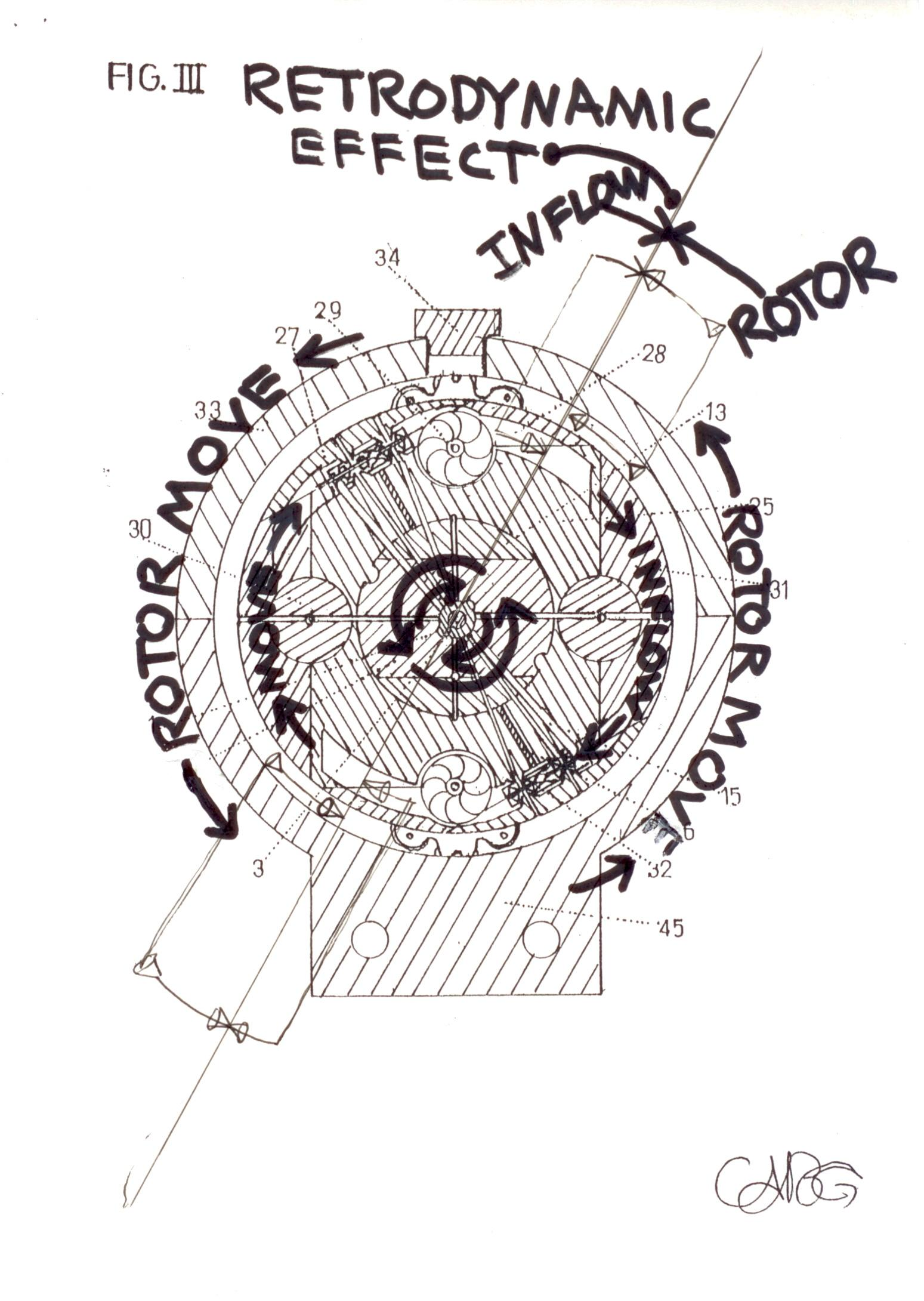Search
Dec 19, 2021Science and Technology
It Can Apply the Newton III Law on an Engine System Device?

Can Physically apply Newton's III 3rd third law of motion, as a cycle system in a Motor Engine Device?

3rd Thermodynamic Law Applicated in a Power-Plant Device. The Reaction at From of the Action. New Torque Power. Its a simple Plus Formula of the Interaction of the Two Powers: 1/Action, + 2/Reaction = Retrodynamic. / For every action there is an equal and opposite reaction. 275L

RETRODYNAMIC Effect - Dextrogiro => vs <= Levogiro Phenomena Effect. / Rotor/RPM VS InFlow/Way. 2dn Thermodynamic Law Applicated in a Power-Plant Device. The Reaction at From of the Action. New Torque Power. Its a simple Pluse Formula of the Interaction of the Two Powers: 1/Accion, + 2/Reaction = Retrodynamic.

"Changing rotation inside a mass makes it possible to change its inertial properties. It is the equation for a jet motion without rejection of any mass.” Albert Einstein.

The formula of the Retrodinamic effect is a simple mathematical summation. What is Dextrogiro plus Levogiro, gives the result of the sum, of what is the total, which is the retrodynamic effect. But keeps doing Exponential.

The logic of creation of an inertial propulsion system is thus: Any motion is rotation ---- Rotation of a matter generates a space-time Torsion ---Torsion of space - time is described by Ricci torsion --- Ricci torsion is an inertial field-----the rest mass of any object is determined by its inertial field---- operating by fields and forces of inertia inside of mass we can create inertial propulsion system which moves according to the equation [m (t) dv/dt =-vdm/dt].

Newton's Third Law of Motion: III. For every action there is an equal and opposite reaction.

It is the sum of both (two) Forces - one to other.

http://stateoftheartnovelinflowtech.blogspot.com

https://www.behance.net/gallery/21019191/Novel-Rotary-Turbo-InFlow-Tech-Gearturbine-Project